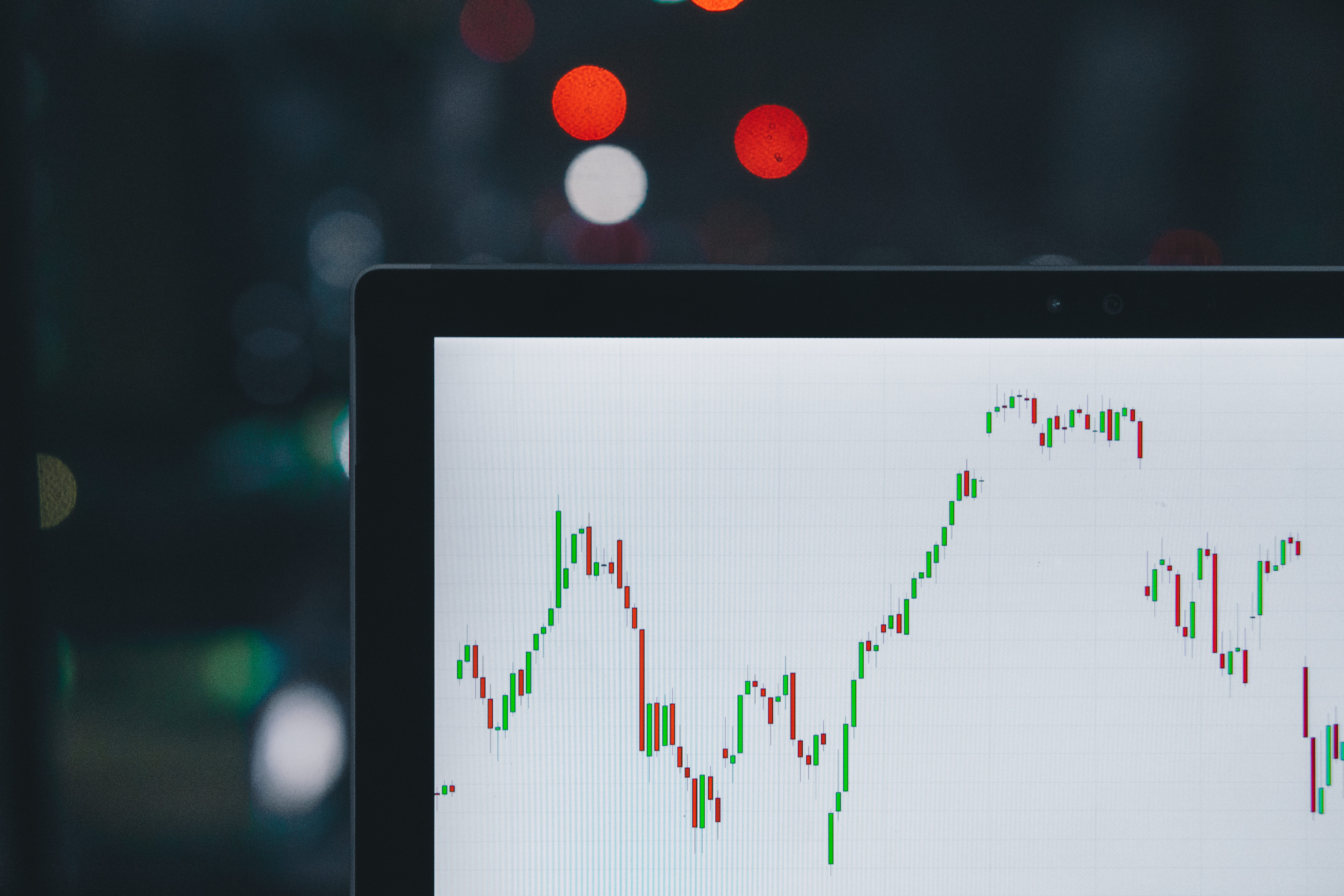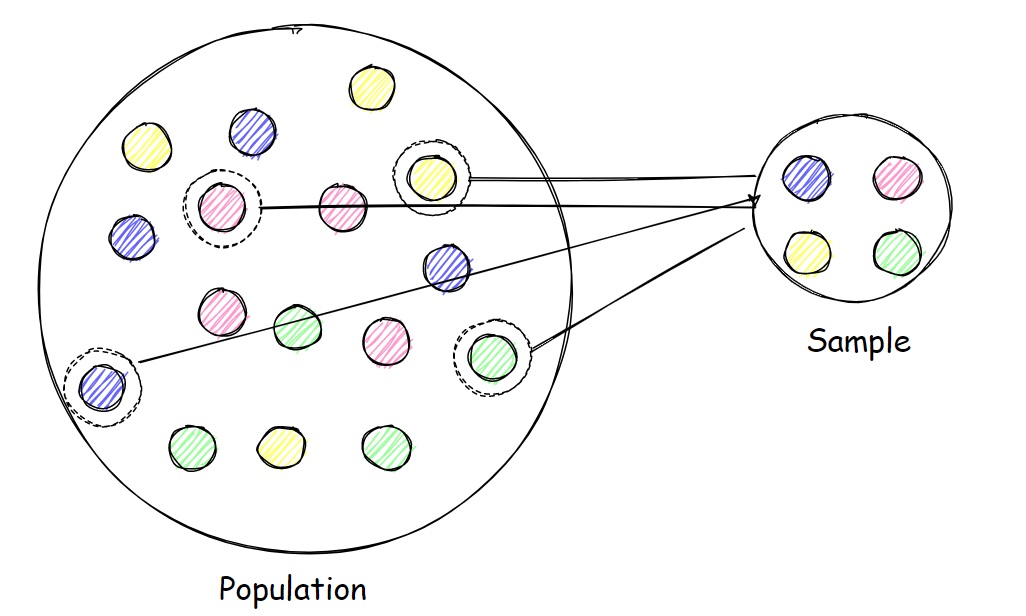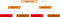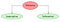# Introduction to Statistics for Data SciencePhoto by M. B. M. on Unsplash

In this day and age, healthy business goes hand in hand with data. Whether you understand it or not, there is no denying that data is at the foundation of any successful company and the business entrepreneurs are aware that digging deeper into data is what will make them tower above the competition. Data science is an ever-evolving field that involves drawing insights from data that is used to drive a business.

# What is statistics? Why is it important?

Statistics is used to describe the principles and methods which are employed in the collection, presentation, analysis, and interpretation of the data. These devices help to simplify the complex data and make it possible for a common man to understand it without much difficulty.

You must have seen the daily frequency chart of coronavirus cases in India. With a simple graphical analysis, one is easily able to interpret what are the total number of new cases on a given date and how the trend has been over a period of time. A simple yet classical example of the importance of statistics in our day-to-day lives.

Statistics is used widely across an array of applications and professions. Any time data are collected and analyzed, statistics are being done. This can range from government agencies to academic research to analyzing investments.

Hence it is vital for anyone who is working with data or remotely working with data, to understand the basics of statistical concepts as it will help them to draw meaningful insights from the data.

There are a few concepts and terminologies to be aware of before proceeding with advanced topics of statistics.

# Sample and PopulationImage by author

The first step of every statistical analysis you perform is to determine the data you are dealing with is a population or a sample.

Population: A population is a collection of all items of interest to our study which has a set of common characteristics. It is denoted by N. N may be finite or infinite. The numbers we obtain when using a population are called parameters.

Let’s say we want to perform a survey of the students’ job prospects studying in your university. What’s the population? You can simply walk into the university and talk to every student, right? Well, the population here consists of not only the students on campus but also the ones at home, exchange students, distant education students, part-time students, etc. As you can see, populations are often hard to define and hard to observe in real life.

Sample: A sample is a subset of the population. The size of a sample is denoted by n, n is always finite. The numbers we obtain working with a sample are called statistics. Now you know why the field we are studying is called statistics.

A sample is much easier to gather, it is less time-consuming and less costly. Time and resources are the main reasons we prefer drawing samples compared to analyzing an entire population. You’ll almost always be working with sample data and make data-driven decisions and inferences based on it.

Since statistical tests are usually based on sample data, samples are key to accurate statistical insights. They have two defining characteristics, Randomness & Representativeness. A sample must be both for an insight to be precise.

A random sample is collected when each member of the sample is chosen strictly by chance. A representative sample is a subset of the population that accurately reflects the members of the entire population. In our example, the best way to achieve it would be to get access to the student database, and contact students at random.

# Types of DataImage by author

Data are the facts and figures that are collected, analyzed, and summarized for presentation and interpretation purposes. Different types of data require different types of statistical and visualization techniques hence before proceeding with any statistical methods it is important to identify what kind of data one is dealing with. Data can be broadly classified into 2 types based on the measurement level as

Quantitative data: These are numerical types of data that can be measured in the form of counts or numbers. Questions like “how much” /“how many” generally give you a quantitative result.

The quantitative data can be further classified into:

• Discrete: Such kind of data can take only certain finite whole number values and can easily be counted. For example, the number of items, grades in an exam, etc. Such values cannot be divided into further levels.
• Continuous: Such kind of data can take an infinite number of values in a given range and thus can take forever to count. For example, your height can be any value in a range of human heights, it could either be 160 cm, or 160.5 cm, or 160.55 cm. In other words, the values can be divided into further granular levels with infinite possibilities. Other examples could include weight, area, distance, time, etc.

Qualitative data: These are non-numerical types of data that describe the qualities or characteristics of an entity. This kind of data is usually obtained using questionnaires, interviews, or observations. They usually provide labels, or names, for categories of items.

The qualitative data can be further classified into:

• Nominal: This type of data is used to group variables into categories, that do not follow any order, they are just provided “names” or “labels”. For example, names of different types of cars, countries, different colors, etc.
• Ordinal: This type of data is used to group variables into categories, that strictly follow a certain order. For example, education levels, rankings, Likert scale, etc.

# Types of Statistics

Statistics can be broadly categorized into 2 types:Image by author

# Descriptive Statistics

As the name suggests, descriptive statistics is used to describe/summarize the data. Suppose a professor computes an average grade for one history class. Because statistics describe the performance of that one class but do not make a generalization about several classes, we can say that the professor is using descriptive statistics. Graphs, tables, and charts that display data so that they are easier to understand are all examples of descriptive statistics.

Descriptive statistics is usually analyzed with the support of measures of central tendency, which mainly comprises of statistical tools such as mean, median, and mode; measures of dispersion which comprises of range, inter-quartile range, standard deviation, variance, and coefficient of variation for a single variable and covariance and correlation when we want to describe a relationship between two variables.

# Inferential Statistics

Now suppose that the history professor decides to use the average grade achieved by one history class to estimate the average grade achieved in all ten sections of the same history course. The process of estimating this average grade would be a problem in inferential statistics.

Infer means “to deduce or conclude (something) from evidence and reasoning rather than from explicit statements”(source). As we know we can’t always deal with population, instead, we use samples from the population to understand the characteristics of the population. Inferential statistics comprises methods that are used to draw conclusions about the characteristics of a population from the characteristics of a sample and to decide how certain they can be of the reliability of those conclusions.

These methods rely on probability theory and distributions, in particular, to predict population values based on sample data. Hypothesis testing, various statistical tests like z-test, t-test, ANOVA, confidence interval, regression analysis are some of the methods that help in drawing insights about a population with the help of samples.

I will be providing a separate article giving a detailed overview of descriptive and inferential statistical methods in my upcoming articles so stay tuned. :)

# Conclusion

To summarize, we have understood that

1. Statistics are used widely across an array of applications and professions, right from research to the corporate world in every domain known to mankind to perform critical analysis.
2. We must understand the kind of data we are dealing with to make the most use out of it.
3. There are two types of statistical methods, descriptive and inferential, and depending on our requirements we can make use of methods in them to derive meaningful insights.

Happy Learning! 🙂

Data science Enthusiast